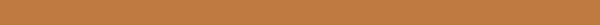# 现代量子力学笔记(七): Shrödinger的画和Heisenberg是一样的(OVA2)

## 结果对比

Schrödinger picture Heisenberg picture
State ket Moving Stationary
Observable Stationary Moving
Base ket Stationary Moving oppositely

## 以上关系的来源

unitary算符在为位移算符或时间演化算符时,会让一个体系的态发生变化. 观察$⟨β|X|α⟩$如何随着该算符变化可以得到: $$(⟨β|U^†)⋅X⋅(U|α⟩)=⟨β|⋅(U^†XU)⋅|α⟩.$$

## Ehrenfest’s Theorem

$$m\frac{d^2}{dt^2}⟨\mathbf{x}⟩=\frac{d⟨\mathbf{p}⟩}{dt}=-⟨∇V(\mathbf{x})⟩.$$